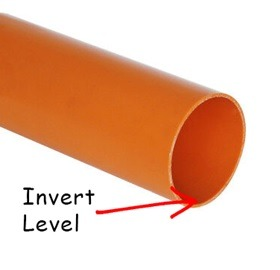# Invert Levels and Falls & Drains in Drainage

## What is the invert level of a pipe?

Invert level is the term given to the level of the bottom of the inside of a drainage pipe or inspection chamber.

Drainage layout drawings usually show cover levels and invert levels of inspection chambers or manholes. The cover level is the finished ground level at a location, measured against a fixed benchmark. The invert level is the inside floor of the pipe or chamber at that location. The invert depth is then calculated from the drawing by subtracting the invert level from the cover level.

For example if the cover level of a chamber is 100.00, and the invert level is 98.78, the invert depth is 1.22 (calculated as 100.00 – 98.78).These measurements need to be considered when laying out any drainage installation, in accordance with pipe falls, connections, & distances from surrounding buildings.

## Falls & Gradients for Drainage

A fall is the term used to denote the distance that a pipe drops vertically relative to its horizontal length - essentially the slope of the pipe.

Fall calculations will be made when planning a project in order to provide adequate flow rates, and ensure solids do not slow down and create a blockage. The fall is defined as the vertical amount the pipe will drop from point A to point B over a certain distance.

### What is a 1 in 40 fall?

A 1 in 40 pipe fall means that for every 40 units of length, the pipe will drop by 1 unit. For example, in a 40 metre run of pipe, the vertical drop of that pipe run will be 1 metre.

It makes no difference what unit of measurement is used.

Pipe fall can also sometimes be described as a gradient. To calculate the gradient, divide the vertical fall by the horizontal length of the pipe run.  So in our 1 in 40 example, the calculation would be (1/40) giving a gradient of 0.025.For example in a 24 metre section of drainage pipe if you had a fall of 0.30 metres and were asked to calculate the gradient:

• Gradient = 0.30 / 24

This can be converted into a gradient written as a ratio or 1

• Gradient = 1 / 0.0125 = 80
• Fall = 1 in 80

The above formula may be rearranged for Fall if the gradient is known: